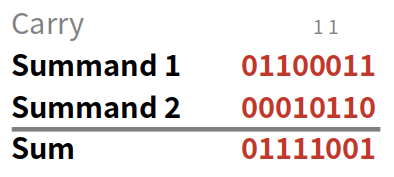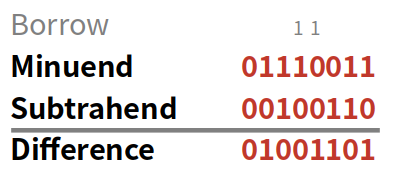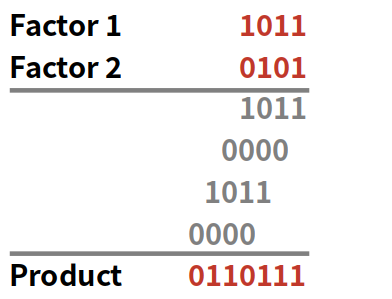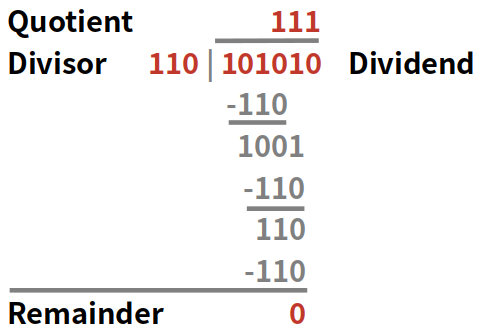# Binary Calculator

Created by Philip Maus
Reviewed by Steven Wooding
Last updated: Mar 11, 2022

The binary calculator makes performing arithmetic binary operations easy. It explains how to calculate binary addition, subtraction, multiplication, and division. The calculator executes all calculations in signed and unsigned representation.

## What are arithmetic binary operations?

Binary numbers are numbers of the base 2, consisting only of the digits 0 and 1. They are a string of bits, which can represent only two logic states: on or off.

Since we are taught arithmetic operations like addition and subtraction based on the decimal system, binary arithmetic operations can seem a bit difficult at first. But by the end of this article, you will see that it's not that demanding! And binary numbers have the great property of allowing operations only limited to this number system, like bit shifts and the bitwise operations AND, OR, and XOR.

Binary numbers can be converted to decimal numbers and back again. We represent negative values of binary numbers in a so-called two's complement signed representation, in which the first bit indicates the sign of the number, 0 meaning negative and 1 positive.

The base for a working binary arithmetic calculator is binary addition. Binary addition works in a very similar way to decimal addition. There are 4 main rules:

• 0 + 0 = 0
• 0 + 1 = 1
• 1 + 0 = 1
• 1 + 1 = 0 (carry 1 to the next bit)

Applying those rules, starting from the rightmost (least significant) bit, will easily add binary numbers. See the example below for a further explanation:## Binary subtraction

Binary subtraction can be executed in two different ways:

• The borrow method is equivalent to the decimal subtraction procedure.
• The complement method replaces the subtrahend by its two's complement and then executes a binary addition, as shown above.

This article only shows the borrow method, for which apply the following rules:

• 0 - 0 = 0
• 0 - 1 = 1 (borrow 1 from the next bit)
• 1 - 0 = 1
• 1 - 1 = 0

Again, we start from the rightmost, least significant bit and work our way to the left. We show how to calculate binary subtraction in the following example:## Binary multiplication

Binary multiplication is very similar to decimal long multiplication, just simpler since we only work with the digits 0 and 1. It is based on the concept of binary addition. Once again, there are four basic rules, but this time we don't need to carry or borrow:

• 0 * 0 = 0
• 0 * 1 = 0
• 1 * 0 = 0
• 1 * 1 = 1

See below an example of the binary arithmetic calculator for multiplication:## Binary division

Binary division strongly follows the decimal long division. The procedure consists of binary multiplication and binary subtraction steps. Starting from the left (most significant bit), it is investigated if the dividends' current digit can be divided by the divisor. If so, a 1 is noted in that position of the quotient; if not, a 0. The remainder of the division process is kept, and the next digit added to it. This procedure is repeated until the rightmost (the least significant bit) is reached.

As an example, let's divide 101010 (the dividend) by 110 (the divisor):Not every binary division works out perfectly with the remainder 0. That's why the binary calculator will present your binary division result with the remainder in the binary and decimal system.

## How to use the binary calculator?

You know how binary addition, subtraction, multiplication, and division work, but those operations can get quite convoluted and confusing for big binary numbers. But don't worry, that's what the binary calculator is there for! So let's take a look at how to use it. As an example, we will subtract the binary equivalent of the decimal number 38 from 115. To convert binary to decimal and reverse, use this tool.

1. Choose your binary representation. That is the number of bits of your input and your result will be represented in. Consider that the operations addition and multiplication will most likely have a result with more significant bits than the input numbers.
• Our bigger input number 1110011 (115 in decimal) has 7 significant bits, and we perform a subtraction, so 8 bits are sufficient.
1. Input your binary numbers in the corresponding fields. Be aware of the order of inputs since subtraction and division are sensitive to this.
• We want to subtract 38 from 115, so the first input is 1110011, and the second input is 100110.
1. Choose the arithmetic binary operation you want to perform.
• For us, this is Subtraction.
1. The result of your arithmetic binary operation is presented in the binary and decimal system.
• Binary: 0100 1101
• Decimal: 77

In case your binary result has a value of 1 on the most significant bit and could be understood as a positive result in unsigned notation or a negative result in signed notation, both results will be displayed.

## FAQ

### How to calculate binary numbers?

Binary numbers allow for the same arithmetic calculations as numbers from the decimal system. Addition, subtraction, multiplication, and division are easily performed with binary numbers. Additionally, bitwise operations like bit shifts, logical AND, OR, and XOR can be executed.

### How do I add binary numbers?

Binary addition works in a similar way to decimal addition. Starting from the least significant bit, add the values of the bit from each summand. If both summands have the value 1 on this bit, carry a 1 in the next higher bit of the result.

### How to calculate binary subtraction?

Binary subtraction can be calculated in two ways:

• The borrow method is similar to the decimal subtraction method. Starting from the least significant bit, subtract the value of the subtrahend from the minuend bit. In case the former is 1 and the latter 0, borrow a 1 from the next higher bit.
• The complement method performs an addition of the minuend and the two's complement of the subtrahend.

### What are the advantages of binary operations?

Binary and bitwise operations are commonly applied due to their advantages in performance and memory needs. This might include registers in processors, embedded systems, data transmission, and video and audio codecs.

### How do I add 3 and 10 in binary?

To add the binary numbers 3 and 8, follow those steps:

1. Convert the numbers from decimal to binary: 0011 and 1010.
2. Add the values of the fourth bit: 1+0 = 1.
3. Add the values of the third bit: 1 + 1 = 0, carry 1 to the next bit.
4. Add the values of the second bit: 0 + 0 + 1 carried = 1.
5. Add the values of the first bit: 0+1=1.
6. Your sum is 1101, or 13 in the decimal system.
Philip Maus
Input data
Binary representation
8-bit
You can write binary numbers with no more than 8 digits. You don't have to input leading zeros.
Number 1
Number 2
Arithmetic operation
Operation
People also viewed…

### Area of a square

How to find the area of a square? What is the formula for the area of a square? 🔷

### Black Hole Collision Calculator

The Black Hole Collision Calculator lets you see the effects of a black hole collision, as well as revealing some of the mysteries of black holes, come on in and enjoy!

### Free fall

Our free fall calculator can find the velocity of a falling object and the height it drops from.

### LCD - Least Common Denominator

With this LCD calculator you can find the lowest common denominator of up to five fractions.